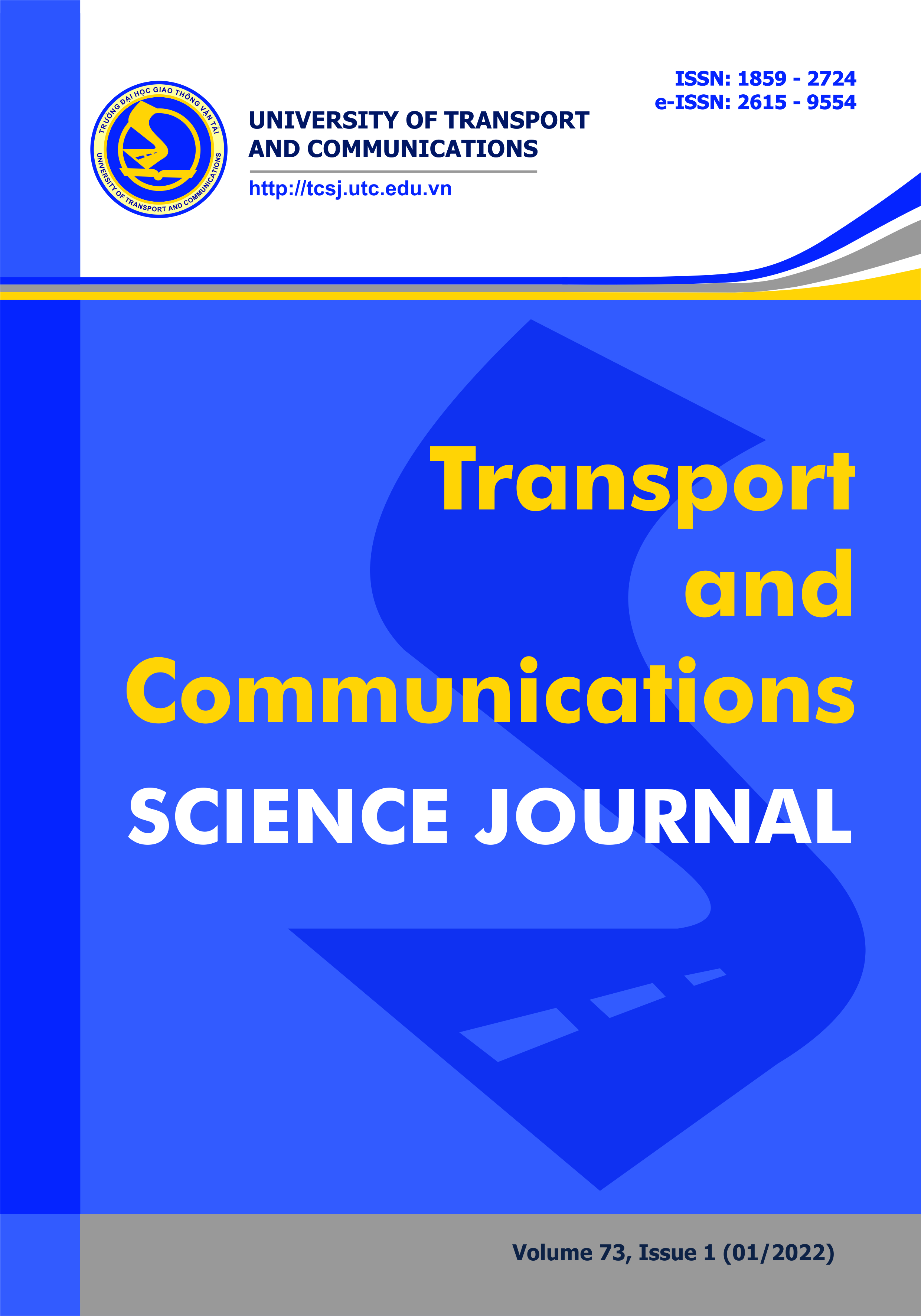#### Algebra of polynomials bounded on some strips

• Du Thi Thu Trang

University of Transport and Communications, No 3 Cau Giay Street, Hanoi, Vietnam
• Nguyen Anh Ngoc

University of Transport and Communications, No 3 Cau Giay Street, Hanoi, Vietnam
Email: dutrang1986@utc.edu.vn
Từ khóa: Positivstellensatze, bounded polynomials, semi-algebraic

#### Tóm tắt

In the present paper, for a finite sequence of single variable polynomials g (x) = (g1(x), g2(x), ..., gn(x)), we study the ring of geometrically bounded elements on a generalized strip Mc(g) in ℝn+1 which is the solution of the system of polynomial inequalities g1(x) £ y1 £ g1(x) + c1, g2(x) £ y2 £ g2(x) + c2 …, gn(x) £ yn £ gn(x) + cn. This ring is shown to be the finitely generated ℝ-algebra ℝ[y1 - g1(x), y2 - g2(x), ..., yn - gn(x)] provided that c = (c1, c2, ..., cn) is a positive vector. However, if c = 0 then this algebra is not finitely generated in general. In particular, we point out that the ring of geometrically bounded elements on ‘a generalized strip’ of the form M(g1, g2) in ℝ2 which is the solution of the polynomial inequality g1(x) £ y £ g2(x) is trivial (i.e., is equal to ℝ) provided that g1(x) is less than g2(x) at infinity. As a consequence, we can describe the ring of geometrically bounded elements on a finite union of disjoint strips

#### Tài liệu tham khảo

. H. V. Ha, T. S. Pham, Genericity in Polynomial Optimization, Series on Optimization and Its Applications, Vol. 3, World Scientific, 2017.
. J. B. Lasserre, Moments, Positive Polynomials and their Applications, Imperial College Press, London, 2009.
. M. Laurent, Sums of squares, moment matrices and optimization over polynomials, in: M. Putinar and S. Sullivant (Eds.), Emerging Applications of Algebraic Geometry. The IMA Volumes in Mathematics and its Applications, Vol. 149, Springer, New York, 2009, pp. 157–270.
. C. Scheiderer, Positivity and sums of squares: a guide to recent results, in: M. Putinar and S. Sullivant (Eds.), Emerging Applications of Algebraic Geometry. The IMA Volumes in Mathematics and its Applications, Vol. 149, Springer, New York, 2009, pp. 271–324.
. T. M. Ho, Trang T. Du, Polynomial optimization on some unbounded semi-algebraic, J. Optim. Theory Appl., 183 (2019) 352–363. https://doi.org/10.1007/s10957-019-01544-5
. K. Schmudgen, The K-moment problem for compact semi-algebraic sets, Math. Ann., 289 (1991) 203–206. https://doi.org/10.1007/BF01446568
. C. Scheiderer, Sums of squares on real algebraic surfaces, Manuscripta Math., 119 (2006) 395–410. https://doi.org/10.1007/s00229-006-0630-5
. H. V. Ha, T. M. Ho, Positive polynomials on nondegenerate basic semi-algebraic sets, Adv. Geom., 16 (2016) 497–510. https://doi.org/10.1515/advgeom-2016-0017
. M. Schweighofer, Iterated rings of bounded elements and generalizations of Schmudgen’s Positivstellensatz, J. Reine Angew. Math., 554 (2003) 19–45. https://doi.org/10.1515/crll.2003.004
. M. Michalska, Algebra of bounded polynomials on a set Zariski closed at infinity cannot be finitely generated, Bull. Sci. Math., 137 (2013) 705–715. https://doi.org/10.1016/j.bulsci.2013.02.006
. P. Mondal, T. Netzer, How fast do polynomials grow on semi-algebraic sets?, J. Algebra, 413 (2014) 320–344. https://doi.org/10.1016/j.jalgebra.2014.05.006
. K. Kurdyka, M. Michalska, S. Spodzieja, Bifurcation values and stability of algebras of bounded polynomials, Adv. Geom., 14 (2014) 631–646. https://doi.org/10.1515/advgeom-2014-0006
. M. Marshall, Polynomials non-negative on a strip, Proc. Amer. Math. Soc., 138 (2010) 1559–1567. https://doi.org/10.1090/S0002-9939-09-10016-3
. V. Powers, Positive polynomials and the moment problem for cylinders with compact cross-section, J. Pure Appl. Algebra, 188 (2004) 217–226. https://doi.org/10.1016/j.jpaa.2003.10.009

### Tải xuống

Chưa có dữ liệu thống kêNhận bài
21/07/2021
Nhận bài sửa
16/11/2021
Chấp nhận đăng
14/01/2022
Xuất bản
15/01/2022
Chuyên mục
Công trình khoa học
Kiểu trích dẫn
Du Thi Thu, T., & Nguyen Anh, N. (1642179600). Algebra of polynomials bounded on some strips. Tạp Chí Khoa Học Giao Thông Vận Tải, 73(1), 90-99. https://doi.org/10.47869/tcsj.73.1.8
Browse